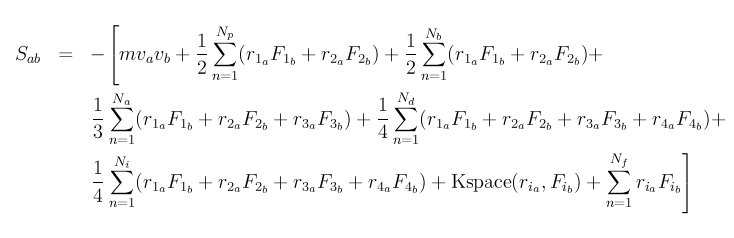# stress per atom calculation

Dear Diaz,
Thank you very much for your help. Actually, You have exactly mentioned to what I have confusing about. if we use Fij*rij which is virial pressure

Stress X (i)= -(m* Vx(i)* Vx(i) + Sum ( Fijrij )) where rij= ri-rj ??? OR Stress X (i)= -(m Vx(i)* Vx(i) + Sum ( rx(i)*Fij+rx(j)*Fij)) ???

Sincerely,Greetings,

That mathematical equation can be figured out by multiplying out Fijrij= Fijri-Fijrj=Fijri+Fji*rj. Summing over all j and i and acknowledging that sum(Fij)=Fi for j not equal to i gives the resulting Stress in terms of total particle force and particle position.

Sincerely,Got it. thank you very much for your clarification. I was really confused.Hi,

I would like to add something on this topic.

For two-body potentials, per atom virial tensor on atom i, W_i, is:

W_i = r_i \otimes F_i (this is a definition and here r_i and F_i are vectors)

Using periodic boundary conditions, it can be transformed to:

W_i = 1/2 * \sum_{j != i} r_ij \otimes F_ij

Here, r_ij = r_i - r_j is the relative position vector, and F_ij is the force vector acting on i due to j.

For many-body potentials, the LAMMPS implementation generalizes these formulas in some way, although I don’t think it is rigorous.

BruceTherefore, the difference between stresses in X and for example y direction is only because of the kinetic part of the equation. tight? the energy or virial part is the same in all stress tensors.

Sincerely,Dear ALL,

Thank you very much for sharing your ideas. I finally found out what is happening. but believe me the formula has been explained very complicated.
Sxx (i) = -( mVx(i) Vx(i) +0.5* SUM over bin ( Fij . ( rx(i) - rx(i) ))
Syy (i) = -( mVy(i) Vy(i) +0.5* SUM over bin ( Fij . ( ry(i) - ry(i) ))

and so one…

Sincerely,Your formulas are still incorrect. The explicit per-atom stress tensor for
(short-ranged) two-body potentials can be written as:

Sxx_i = m_i vx_i * vx_i + 0.5 * sum_j Fx_ij * (x_i - x_j)

Sxy_i = m_i vx_i * vy_i + 0.5 * sum_j Fx_ij * (y_i - y_j)

Sxz_i = m_i vx_i * vz_i + 0.5 * sum_j Fx_ij * (z_i - z_j)

Syx_i = m_i vy_i * vx_i + 0.5 * sum_j Fy_ij * (x_i - x_j)

Best,
Bruce